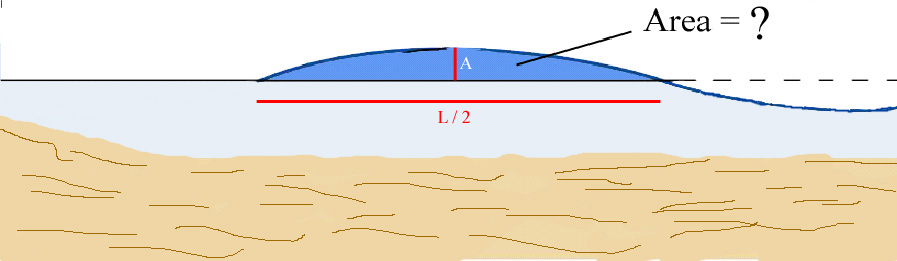Hints:

b.1)If you cannot solve this by calculus, the area is:

A • L  ,

p

where A is the amplitude of the wave and L is the wavelength.

b.2) Calculate the volume of this 1 m wide section, and use the formula for kinetic energy:

m • v2  ,

2

where v is the speed of the wave. Note that 1 m3 of water contains 1000 kg of water!